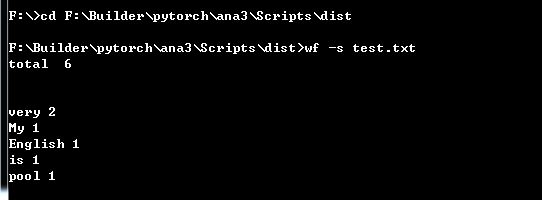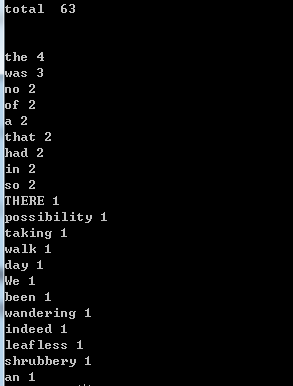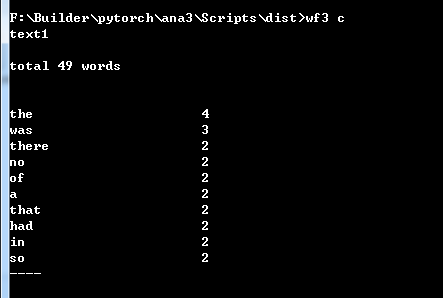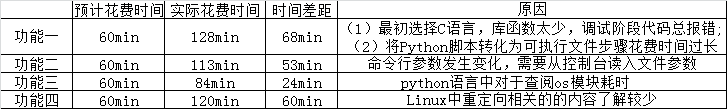# 20200917-2 词频统计

（1） 考查文件读取模块open()函数的使用;
（2） 计算单词个数的时候，将读入的单词用split()函数将单词与符号分隔开;
（3） Python文件转换为可执行文件流程不熟悉，花费了较长时间。

#!/usr/bin/env python3
#-*- coding:utf-8 -*-
import sys
import re
import collections
import operator
import glob
file_name='F:\\研究生\\研究生作业\\构建之法作业\\第二次\\text.txt'
total=0
file=open(file_name,"r")  #open函数打开文件，str为文件名，'r'代表只读
w = line.split(' ')     #空格为分隔符分割每行的单词，并返回字符串列表
total=total + len(w)    #计算单词总数

print ("total ",total)       #总数结果打印
print('\n')
#相同单词出现次数
patt = re.compile("\w+")   #正则表达式
counter = collections.Counter(patt.findall(
))
for word, show in counter.most_common():  #用word变量记录单词，用show变量记录单词出现的频数，返回一个topn列表，其中不对counter.most_common(n)的n指定参数，返回所有元素

print (word, show)（1） 命令行参数发生变化，需要从控制台读入文件参数，进行运算;
（2） 数据量比较大，最初用C语言进行尝试，库函数较少，且遇到数据量较大的文件，就会导致程序崩溃，根据同学建议，换用python语言进行尝试实现了功能。

#!/usr/bin/env python3
#-*- coding:utf-8 -*-
import sys
import re
import collections
import operator
import glob

def funtion_2(file_name):
#file_name='F:\\研究生\\研究生作业\\构建之法作业\\第二次\\text.txt'
total=0
file=open(file_name,"r")  #open函数打开文件，str为文件名，'r'代表只读
w = line.split(' ')     #空格为分隔符分割每行的单词，并返回字符串列表
total=total + len(w)    #计算单词总数

print ("total ",total)       #总数结果打印
print('\n')
#相同单词出现次数
patt = re.compile("\w+")   #正则表达式
counter = collections.Counter(patt.findall(
))
for word, show in counter.most_common():  #用word变量记录单词，用show变量记录单词出现的频数，返回一个topn列表，其中不对counter.most_common(n)的n指定参数，返回所有元素

print (word, show)

a=input("输入文件路径：\n")
funtion_2(a)
m=input("输入回车结束")（1） 和功能二命令行区别在于，输入命令行参数的时候，输入的是文件夹，而不是文件处理和功能二命令行参数之间的区别;
（2） 需要根据文件夹的名字找到文件，并且进行词频统计。

#!/usr/bin/env python3
#-*- coding:utf-8 -*-
import sys
import re
import collections
import operator
import glob
#str=str+ '.txt'                                                     #用于读取文件并返回分词之后的单词列表
f = open(str,'r',-1,'utf-8','ignore',None,True,None)
text = tet.replace('\n',' ').replace('.',' ').replace(',',' ').\
replace('!',' ').replace('\\',' ').replace('#',' ').\
replace('[',' ').replace(']',' ').replace(':',' ').\
replace('?',' ').replace('-',' ').replace('\'',' ').\
replace('\"',' ').replace('(',' ').replace(')',' ').\
replace('—',' ').replace(';',' ').split()
count_dict = {}
for str in text:
if str in count_dict.keys():
count_dict[str] = count_dict[str] + 1
else:
count_dict[str] = 1
count_list=sorted(count_dict.items(),key=lambda x:x,reverse=True)
f.close()
return count_list                                                          #返回的分词列表
#对分完词之后的列表进行计算total、词频、输出等操作
def get_words(argv,flag):
if len(argv) == 2:                                                         #如果有两个命令行参数
try:
opts, args = getopt.getopt(argv,"sh",["ifile","ofile"])
except getopt.GetoptError:
print("test.py -i <inputfile> -o <outputfile>")
sys.exit(2)
for opt,arg in opts:
if opt == "-s":                                                    #如果第一个参数是-s
num = len(list)
print('total',num)
print('\n')
for word in list:
print('{:20s}{:>5d}{}'.format(word,word,'\n'))
elif opt == "-h":
elif len(argv) == 1:                                                       #如果有一个命令行参数
pattern = re.compile('.+\.txt')
folder_name = argv[-1]
m = re.findall(pattern,folder_name)
if len(m) != 0:                                                          #参数以.txt结尾，为文件名，直接执行词频操作
if flag == 0:                                                        #标志位为0，把所有单词都列出来
print('total',len(list))
print('\n')
for item in list:
print('{:20s}{:>5d}{}'.format(item,item,'\n'))
else:                                                                #标志位不为0，只列出前十
print('total',len(list), 'words')
print('\n')
if len(list) > 10:
for i in range(10):
print('{:20s}{:>5d}'.format(list[i],list[i]))
else:                                                            #如果本身不超过10个单词量，则列出所有单词即可
for item in list:
print('{:20s}{:>5d}'.format(item,item))
else:
os.chdir(folder_name)                                                #文件夹操作
filename_list = os.listdir()
for file_name in filename_list:
print(file_name[:-4] + '\n')
file_list = [file_name]
get_words(file_list,1)                                           #得到文件名，进行递归操作，标志位置1，说明要取前10
print('----\n')

def main(argv):
get_words(argv,0)

if __name__ == "__main__":
main(sys.argv[1:])posted @ 2020-09-23 08:31  阳光使人温暖  阅读(108)  评论(0编辑  收藏